# How to use Excel SUMIFS and SUMIF with multiple criteria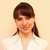by , updated on

This tutorial explains the difference between the SUMIF and SUMIFS functions in terms of their syntax and usage, and provides a number of formula examples to sum values with multiple AND / OR criteria in Excel 365, 2021, 2019, 2016, 2013, 2010, and lower.

As everyone knows, Microsoft Excel provides an array of functions to perform various calculations with data. A few articles ago, we explored COUNTIF and COUNTIFS, which are designed for counting cells based on a single condition and several conditions, respectively. Last week we covered Excel SUMIF that adds values meeting the specified criteria. Now it's time to go over the plural version of SUMIF - Excel SUMIFS that allows summing values by multiple criteria.

Those who are familiar with the SUMIF function might think that converting it to SUMIFS takes just an extra "S" and a few additional criteria. This would seem quite logical… but "logical" it's not always the case when dealing with Microsoft : )

## Excel SUMIF function - syntax & usage

The SUMIF function is used to conditionally sum values based on a single criteria. We discussed the SUMIF syntax in detail in the previous article, and here's just a quick refresher.

SUMIF(range, criteria, [sum_range])
• range - the range of cells to be evaluated by your criteria, required.
• criteria - the condition that must be met, required.
• sum_range - the cells to sum if the condition is met, optional.

As you see, the syntax of the Excel SUMIF function allows for one condition only. And still, we say that Excel SUMIF can be used to sum values with multiple criteria. How can that be? By adding the results of several SUMIF functions and by using SUMIF formulas with array criteria, as demonstrated in the examples that follow.

## Excel SUMIFS function - syntax & usage

You use SUMIFS in Excel to find a conditional sum of values based on multiple criteria. The SUMIFS function was introduced in Excel 2007 and is available in all subsequent versions of Excel 2010, 2013, 2016, 2019, 2021, and Excel 365.

Compared to SUMIF, the SUMIFS syntax is a little bit more complex:

SUMIFS(sum_range, criteria_range1, criteria1, [criteria_range2, criteria2], …)

The first 3 arguments are mandatory, additional ranges and their associated criteria are optional.

• `sum_range` - one or more cells to sum, required. This can be a single cell, a range of cells or a named range. Only cells with numbers are summed; blank and text values are ignored.
• `criteria_range1` - the first range to be evaluated by the associated criteria, required.
• `criteria1` - the first condition that must be met, required. You can supply the criteria in the form of a number, logical expression, cell reference, text or another Excel function. For example you can use criteria such as 10, ">=10", A1, "cherries" or TODAY().
• `criteria_range2, criteria2, …` - these are additional ranges and criteria associated with them, optional. You can use up to 127 range/criteria pairs in SUMIFS formulas.

Notes:

• For a SUMIFS formula to work correctly, all the criteria_range arguments must have the same dimension as sum_range, i.e. the same number of rows and columns.
• The SUMIFS function works with AND logic, meaning that a cell in the sum range is summed only if it meets all of the specified criteria, i.e. all the criteria are true for that cell.

## Basic SUMIFS formula

And now, let's have a look at the Excel SUMIFS formula with two conditions. Suppose, you have a table listing the consignments of fruit from different suppliers. You have the fruit names in column A, suppliers' names in column B, and quantity in column C. What you want is to find out a sum of amounts relating to a given fruit and supplier, e.g. all apples supplied by Pete.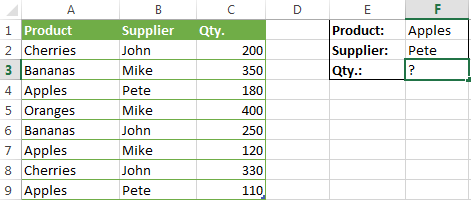When you're learning something new, it's always a good idea to start with simple things. So, to begin with, let's define all the arguments for our SUMIFS formula:

• sum_range - C2:C9
• criteria_range1 - A2:A9
• criteria1 - "apples"
• criteria_range2 - B2:B9
• criteria2 - "Pete"

Now assemble the above parameters, and you will get the following SUMIFS formula:

`=SUMIFS(C2:C9, A2:A9, "apples", B2:B9, "Pete")`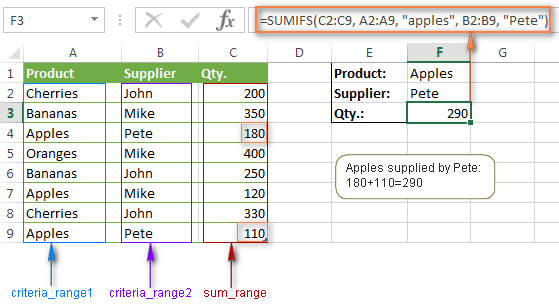To refine the formula further, you can replace the text criteria "apples" and "Pete" with cell references. In this case, you won't have to change the formula to calculate the quantity of other fruit from a different supplier:

`=SUMIFS(C2:C9, A2:A9, F1, B2:B9, F2)`

Note. Both the SUMIF and SUMIFS functions are case-insensitive by nature. To get them to recognize the text case, please see Case-sensitive SUMIF and SUMIFS formula in Excel.

## SUMIF vs. SUMIFS in Excel

Since the aim of this tutorial is to cover all possible ways to sum values by several conditions, we will discuss formula examples with both functions - Excel SUMIFS and SUMIF with multiple criteria. To use them correctly, you need to clearly understand what these two functions have in common and in what way they are different.

While the common part is clear (similar purpose and parameters), the differences are not so obvious, though very essential.

There are 4 major differences between SUMIF and SUMIFS:

1. Number of conditions. SUMIF can evaluate just one condition at a time while SUMIFS can check for multiple criteria.
2. Syntax. With SUMIF, the sum_range is the last and optional argument - if not defined, the values in the range argument are summed. With SUMIFS, sum_range is the first and required argument.
3. Size of ranges. In SUMIF formulas, sum_range does not necessarily have to be of the same size and shape as range, as long as you have the top left cell right. In Excel SUMIFS, each criteria_range must contain the same number of rows and columns as the sum_range argument.

For example, SUMIF(A2:A9,F1,C2:C18) will return the correct result because the leftmost cell in the sum_range argument (C2) is right. So, Excel will make the correction automatically and include as many columns and rows in sum_range as there are in range.

A SUMIFS formula with unequally sized ranges will return a #VALUE! error.

4. Availability. SUMIF is available in all Excel versions, from 365 through 2000. SUMIFS is available in Excel 2007 and higher.

Alright, enough strategy (i.e. theory), let's get into the tactics (i.e. formula examples : )

## How to use SUMIFS in Excel - formula examples

A moment ago, we discussed a simple SUMIFS formula with two text criteria. In the same manner, you can use Excel SUMIFS with multiple criteria expressed by numbers, dates, logical expressions, and other Excel functions.

### Example 1. Excel SUMIFS with comparison operators

In our fruit suppliers table, suppose, you want to sum all deliveries by Mike with Qty. 200 or more. To do this, you use the comparison operator "greater than or equal to" (>=) in criteria2 and get the following SUMIFS formula:

`=SUMIFS(C2:C9,B2:B9,"Mike",C2:C9,">=200")`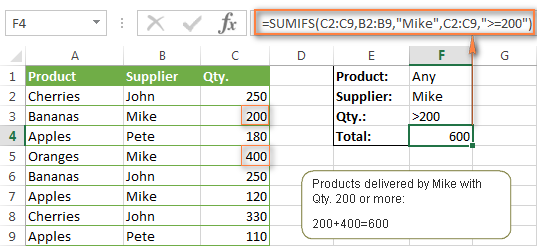Note. Please pay attention that in Excel SUMIFS formulas, logical expressions with comparison operators should always be enclosed in double quotes ("").

We covered all possible comparison operators in detail when discussing Excel SUMIF function, the same operators can be used in SUMIFS criteria. For example, the following formula with return the sum of all values in cells C2:C9 that are greater than or equal to 200 and less than or equal to 300.

`=SUMIFS(C2:C9, C2:C9,">=200", C2:C9,"<=300")`

### Example 2. Using Excel SUMIFS with dates

In case you want to sum values with multiple criteria based on the current date, use the TODAY() function in your SUMIFS criteria, as demonstrated below. The following formula sums values in column D if a corresponding date in column C falls within the last 7 days, including today:

`=SUMIFS(D2:D10, C2:C10,">="&TODAY()-7, C2:C10,"<="&TODAY())`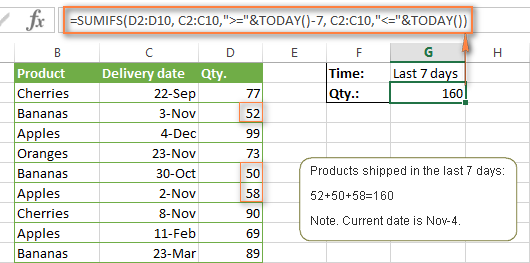Note. When you use another Excel function together with a logical operator in the criteria, you have to use the ampersand (&) to concatenate a string, for example "<="&TODAY().

In a similar fashion, you can use the Excel SUMIF function to sum values in a given date range. For example, the following SUMIFS formula adds the values in cells C2:C9 if a date in column B falls between 1-Oct-2014 and 31-Oct-2014, inclusive.

`=SUMIFS(C2:C9, B2:B9, ">=10/1/2014", B2:B9, "<=10/31/2014")`

The same result can be achieved by calculating the difference of two SUMIF functions, as demonstrated in this example - How to use SUMIF to sum values in a given date range. However, Excel SUMIFS is much easier and more understandable, isn't it?

### Example 3. Excel SUMIFS with blank and non-blank cells

When analyzing reports and other data, you may often need to sum values corresponding either to empty or non-empty cells.

Criteria Description Formula Example
Blank cells "=" Sum values corresponding to blank cells that contain absolutely nothing - no formula, no zero length string. =SUMIFS(C2:C10, A2:A10, "=", B2:B10, "=")

Sum values in cells C2:C10 if the corresponding cells in columns A and B are absolutely empty.

"" Sum values corresponding to "visually" blank cells including those that contain empty strings returned by some other Excel function (for example, cells with a formula like =""). =SUMIFS(C2:C10, A2:A10, "", B2:B10, "")

Sum values in cells C2:C10 with the same conditions as the above formula, but includes empty strings.

Non-blank cells "<>" Sum values corresponding to non-empty cells, including zero length strings. =SUMIFS(C2:C10, A2:A10, "<>", B2:B10, "<>")

Sum values in cells C2:C10 if the corresponding cells in columns A and B are not empty, including cells with empty strings.

SUM-SUMIF
or
SUM / LEN
Sum values corresponding to non-empty cells, not including zero length strings. =SUM(C2:C10) - SUMIFS(C2:C10, A2:A10, "", B2:B10, "")

=SUM((C2:C10) * (LEN(A2:A10)>0)*(LEN(B2:B10)>0))

Sum values in cells C2:C10 if the corresponding cells in columns A and B are not empty, cells with zero length strings are not included.

And now, let's see how you can use a SUMIFS formula with "blank" and "non-blank" criteria on real data.

Suppose, you have an order date in column B, delivery date in column C and Qty. in column D. How do you find the total of products that have not been delivered yet? That is, you want to know the sum of values corresponding to non-empty cells in column B and empty cells in column C.

The solution is to use the SUMIFS formula with 2 criteria:

`=SUMIFS(D2:D10, B2:B10,"<>", C2:C10,"=")`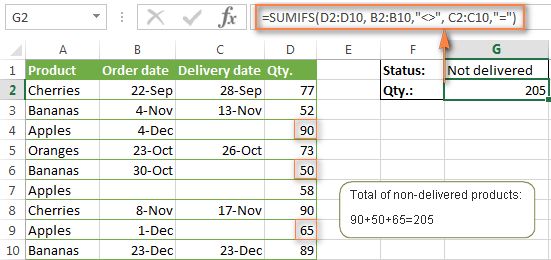## Using Excel SUMIF with multiple OR criteria

As noted in the beginning of this tutorial, the SUMIFS function is designed with AND logic. But what if you need to sum values with multiple OR criteria, i.e. when at least one of the conditions is met?

### Example 1. SUMIF + SUMIF

The simplest solution is to sum the results returned by several SUMIF functions. For example, the following formula demonstrates how to find the total of products delivered by Mike and John:

`=SUMIF(C2:C9,"Mike",D2:D9) + SUMIF(C2:C9,"John",D2:D9)`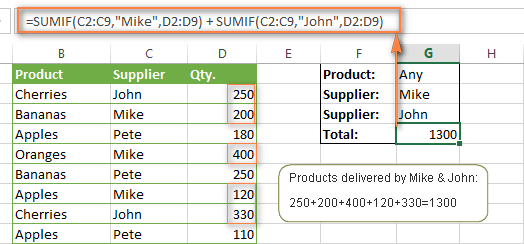As you see, the first SUMIF function adds the quantities corresponding to "Mike", the other SUMIF function returns the amounts relating to "John" and then you add these 2 numbers.

### Example 2. SUM & SUMIF with an array argument

The above solution is very simple and may get the job done quickly when there are only a couple of criteria. But a SUMIF + SUMIF formula may grow up enormously if you want to sum values with multiple OR conditions. In this case, a better approach is using an array criteria argument in the SUMIF function. Let's examine this approach now.

You can start by listing all of your conditions separated by commas and then enclose the resulting comma-separated list in {curly brackets}, which is technically called an array.

In the previous example, if you want to sum the products delivered by John, Mike and Pete, your array criteria will look like {"John","Mike","Pete"}. And the complete SUMIF function is `SUMIF(C2:C9, {"John","Mike","Pete"} ,D2:D9)`.

The array argument consisting of 3 values forces your SUMIF formula to return three separate results, but since we write the formula in a single cell, it would return the first result only - i.e. the total of products delivered by John. To get this array-criteria approach to work, you have to use one more little trick - enclose your SUMIF formula in a SUM function, like this:

`=SUM(SUMIF(C2:C9, {"John","Mike","Pete"} , D2:D9))`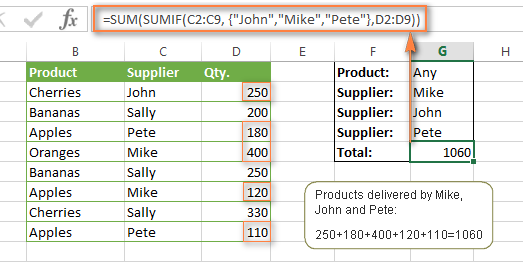As you see, an array criteria makes the formula much more compact compared to SUMIF + SUMIF, and lets you add as many values as you like in the array.

This approach works with numbers as well as with text values. For instance, if instead of the suppliers' names in column C, you had supplier IDs like 1, 2, 3 etc., then your SUMIF formula would look similar to this:

`=SUM(SUMIF(C2:C9, {1,2,3} , D2:D9))`

Unlike text values, numbers needn't be enclosed in double quotes in array arguments.

### Example 3. SUMPRODUCT & SUMIF

In case, your preferred way is to list the criteria in some cells rather that specify them directly in the formula, you can use SUMIF in conjunction with the SUMPRODUCT function that multiplies components in the given arrays, and returns the sum of those products.

`=SUMPRODUCT(SUMIF(C2:C9, G2:G4, D2:D9))`

Where G2:G4 are the cells containing your criteria, the suppliers' names in our case, as illustrated in the screenshot below.

But of course, nothing prevents you from listing the values in an array criteria of your SUMIF function if you want to:

`=SUMPRODUCT(SUMIF(C2:C9, {"Mike","John","Pete"}, D2:D9))`

The result returned by both formulas will be identical to what you see in the screenshot: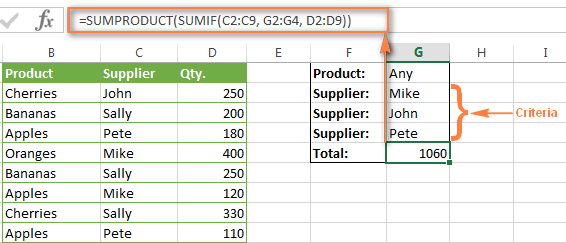## Excel SUMIFS with multiple OR criteria

If you want to conditionally sum values in Excel not simply with multiple OR conditions, but with several sets of conditions, you will have to use SUMIFS instead of SUMIF. The formulas will be very similar to what we've just discussed.

As usual, an example might help to illustrate the point better. In our table of fruit suppliers, let's add the Delivery Date (column E) and find the total quantity delivered by Mike, John and Pete in October.

### Example 1. SUMIFS + SUMIFS

The formula produced by this approach includes a lot of repetition and looks cumbersome, but it is easy to understand and, most importantly, it works : )

```=SUMIFS(D2:D9,C2:C9, "Mike", E2:E9,">=10/1/2014", E2:E9, "<=10/31/2014") + SUMIFS(D2:D9, C2:C9, "John", E2:E9, ">=10/1/2014", E2:E9, "<=10/31/2014") + SUMIFS(D2:D9, C2:C9, "Pete", E2:E9, ">=10/1/2014" ,E2:E9, "<=10/31/2014") ```

As you see, you write a separate SUMIFS function for each of the suppliers and include two conditions - equal to or greater than Oct-1 (">=10/1/2014",) and less than or equal to Oct 31 ("<=10/31/2014"), and then you sum the results.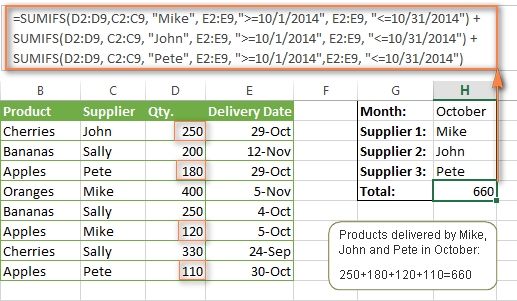### Example 2. SUM & SUMIFS with an array argument

I've tried to explain the essence of this approach in the SUMIF example, so now we can simply copy that formula, change the order of arguments (as you remember it is different in SUMIF and SUMIFS) and add additional criteria. The resulting formula is more compact than SUMIFS + SUMIFS:

`=SUM(SUMIFS(D2:D9,C2:C9, {"Mike", "John", "Pete"}, E2:E9,">=10/1/2014", E2:E9, "<=10/31/2014"))`

The result returned by this formula is exactly the same as you see in the screenshot above.

### Example 3. SUMPRODUCT & SUMIFS

As you remember, the SUMPRODUCT approach differs from the previous two in the way that you enter each of your criteria in a separate cell rather that specify them directly in the formula. In case of several criteria sets, the SUMPRODUCT function won't suffice and you will have to employ ISNUMBER and MATCH as well.

So, assuming that the Supplies Names are in cells H1:H3, Start Date is in cell H4 and End Date in cell H5, our SUMPRODUCT formula takes the following shape:

`=SUMPRODUCT(--(E2:E9>=H4), --(E2:E9<=H5), --(ISNUMBER(MATCH(C2:C9, H1:H3,0))), D2:D9)`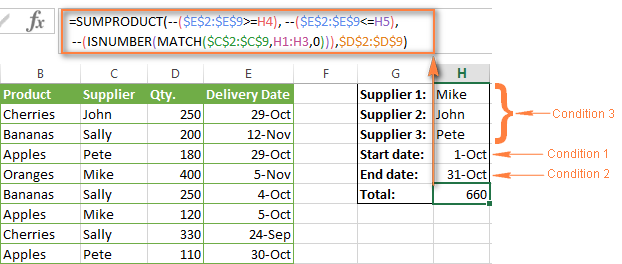Many people wonder why use double dash (--) in SUMPRODUCT formulas. The point is that Excel SUMPRODUCT ignores all but numeric values, while the comparison operators in our formula return Boolean values (TRUE / FALSE), which are non-numeric. To convert these Boolean values to 1's and 0's, you use the double minus sign, which is technically called the double unary operator. The first unary coerces TRUE/FALSE to -1/0, respectively. The second unary negates the values, i.e. reverses the sign, turning them into +1 and 0, which the SUMPRODUCT function can understand.

I hope the above explanation makes sense. And even if it doesn't, just remember this rule of thumb - use the double unary operator (--) when you are using comparison operators in your SUMPRODUCT formulas.

## Using Excel SUM in array formulas

As you remember, Microsoft implemented the SUMIFS function in Excel 2007. If someone still uses Excel 2003, 2000 or earlier, you will have to use a SUM array formula to add values with multiple AND criteria. Naturally, this approach works in modern versions of Excel 2013 - 2007 too, and can be deemed an old-fashioned counterpart of the SUMIFS function.

In the SUMIF formulas discussed above, you have already used array arguments, but an array formula is something different.

### Example 1. Sum with multiple AND criteria in Excel 2003 and earlier

Let's get back to the very first example where we found out a sum of amounts relating to a given fruit and supplier:As you already know, this task is easily accomplished using an ordinary SUMIFS formula:
`=SUMIFS(C2:C9, A2:A9, "apples", B2:B9, "Pete")`

And now, let's see how the same task can be fulfilled in early "SUMIFS-free" versions of Excel. First off, you write down all the conditions that should be met in the form of range="condition". In this example, we have two range/condition pairs:

Condition 1: A2:A9="apples"

Condition 2: B2:B9="Pete"

Then, you write a SUM formulas that "multiplies" all of your range/condition pairs, each enclosed in brackets. The last multiplier is the sum range, C2:C9 in our case:

`=SUM((A2:A9="apples") * ( B2:B9="Pete") * ( C2:C9))`

As illustrated in the screenshot below, the formula perfectly works in the latest Excel 2013 version.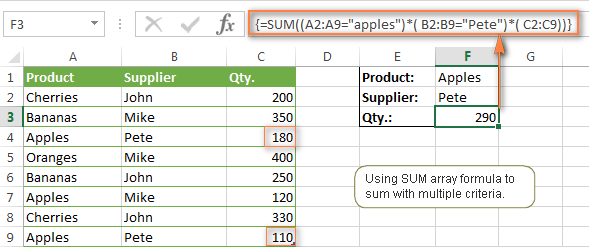Note. When entering any array formula, you must press Ctrl + Shift + Enter. Once you do this, your formula gets enclosed in {curly braces}, which is a visual indication that an array formula is entered correctly. If you try typing the braces manually, your formula will be converted to a text string, and it won't work.

### Example 2. SUM array formulas in modern Excel versions

Even in modern versions of Excel, the power of the SUM function should not be underestimated. The SUM array formula is not simply gymnastics of the mind, but has a practical value, as demonstrated in the following example.

Suppose, you have two columns, B and C, and you need to count how many times column C is greater than column B, when a value in column C is greater or equal to 10. An immediate solution that comes to mind is using the SUM array formula:

`=SUM((C1:C10>=10) * (C1:C10>B1:B10))`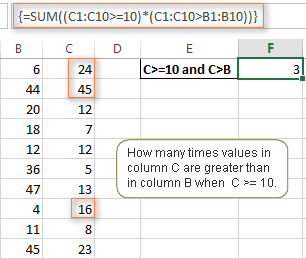Don't see any practical application to the above formula? Think about it in another way : )

Suppose, you have the orders list like shown in the screenshot below and you want to know how many products have not been delivered in full by a given date. Translated into Excel's language, we have the following conditions:

Condition 1: A value in column B (Ordered items) is greater than 0

Condition 2: A value in column C (Delivered) in less than in column B

Condition 3: A date in column D (Due date) is less than 11/1/2014.

Putting the three range/condition pairs together, you get the following formula:

`=SUM((B2:B10>=0)*(B2:B10>C2:C10)*(D2:D10<G2))`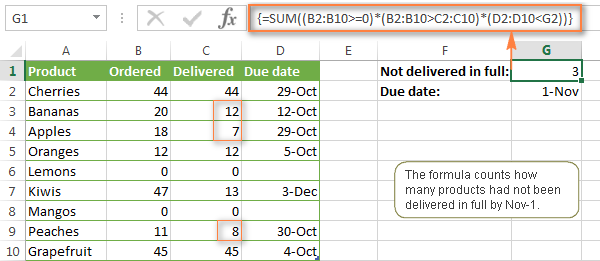Well, the formula examples discussed in this tutorial have only scratched the surface of what Excel SUMIFS and SUMIF functions can really do. But hopefully, they have helped pointing you in the right direction and now you can sum values in your Excel workbooks no matter how many intricate conditions you have to consider.